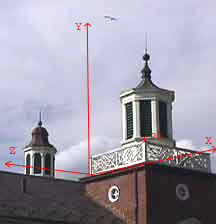No.

# RepresentationYou can think about the points, lines, and plane figures that compose the 3D scene without using a coordinate system. In fact, that is what we have been doing. But in computer graphics we need to represent these objects, somehow, and to manipulate them. To do this, we need a method to represent such objects.

Pick a convenient point in the 3D scene and call it the origin. Now define three lines called X, Y, and Z that go through the origin. Usually the three lines are perpendicular to each other. Each line is called an axis.

Pick a positive direction for each line. There are several choices here, but let's make the choice shown in the picture.

Now each point of the X axis is a unique distance (positive, negative, or zero) from the origin. For example, the corner of the building that lies on the X axis is at a distance of 8.0 feet (or so) from the origin.

### QUESTION 8:

The origin is at a height of zero. About how high is the end point of the closest spike?